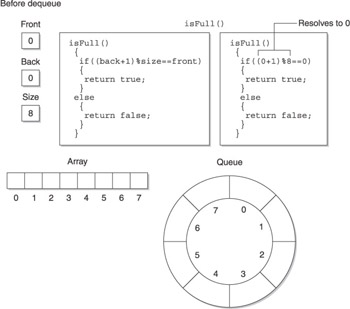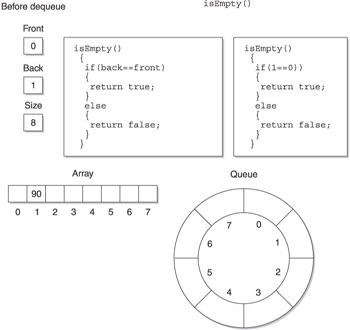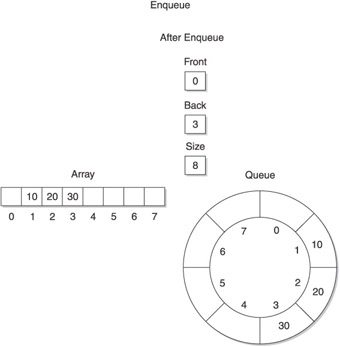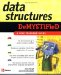# Queues Using an Array in C

## Queues Using an Array in C++

Now that you understand how queues work with an array, it is time to create a real queue. In this section, you ll create a queue using C++. You ll see the Java version of this program later in this chapter.

The C++ queue program is organized into three files: the queue.h file, the queue.cpp file, and the queueProgram.cpp file. The queue.h file, shown next , sets the default size of the array and defines the Queue class. The Queue class declares size , front , and back attributes that store the array size and the index of the front and back of the queue. The Queue class also declares a pointer that will point to the array. In addition to these, the Queue class defines a set of member functions that manipulate the queues. These are explained later in this section.

` //queue.h #define DEFAULT_SIZE 8 class Queue{  private:  const int size;  int front;  int back;  int* values;  public:  Queue(int size = DEFAULT_SIZE);  virtual ~Queue();  bool isFull();  bool isEmpty();  void enqueue(int);  int dequeue(); }; `

The queue.cpp file contains the implementation of the member functions for the Queue class. There are six member functions defined in this file: Queue() , ~Queue() , isFull() , isEmpty() , enqueue() , and dequeue() .

The Queue() member function is a constructor, which is passed the size of the array when an instance of the Queue class is declared. If the constructor is called with no parameters, then the default size is used; otherwise , the value passed to the constructor is used. The value of the array size is assigned to the attribute size by the first statement within the constructor.

The second statement uses the new operator to declare an array of integers whose size is determined by the size passed to the constructor. The new operator returns a pointer to the array, which is assigned to the values pointer. The last two statements in the constructor initialize the front and back attributes to zero.

The ~Queue() member function is the destructor and uses the delete operator to remove the array from memory when the instance of the Queue class goes out of scope.

The isFull() member function (see Figure 5-4) determines if there is room available in the queue by comparing the calculated value of the back of the queue with the value of the front of the queue, as in shown Figure 5-4. Notice that the expression that calculates the back of the queue is very similar to the expression in the enqueue process (see the Enqueue section of this chapter), and both produce the same result. The queue is full when the back index is 1 behind the front. Placing another element in the queue would overwrite the front element and corrupt the queue. The modulus operator is used again to make this a circular queue, so when you re at element 7 on the back, the next element to look at is element 0.Figure 5-4: The isFull() member function determines if there is room to place another item on the back of the queue.

The isFull() member function is called by the enqueue() member function before an attempt is made to place a value on the back of the queue. The isFull() member function returns a true if no more room is available in the queue or a false if there is room available.

The isEmpty() member function determines (see Figure 5-5) if the queue is empty by comparing the back and front variables . If they have the same values, a true is returned; otherwise, a false is returned. The isEmpty() member function is called within the dequeue() member function before it attempts to remove the front item from the queue.Figure 5-5: The isEmpty() member function determines if the queue contains any values.

The enqueue() member function places an item at the back of the queue, as described in the Enqueue section of this chapter. The enqueue() member function is passed the value that is to be placed in the queue. However, before doing so, the isFull() member function is called to determine if there is room available in the queue. Notice in the following example that the isFull() member function is called as the condition expression of the if statement. Also notice that the not operator reverses the bool value returned by the isFull() method. That is, a false is returned by the isFull() member function if room is available in the queue. The condition expression in the if statement reverses this logic to true so that statements within the if statement execute to place the new item on the back of the queue.

The dequeue() member function removes an item from the queue and returns that item to the statement within the program that calls the dequeue() member function. However, the isEmpty() member function is called in the condition expression of the if statement within the dequeue() member function, as shown in the next code listing.

The not operator in this expression reverses the logic returned by the isEmpty() member function. The isEmpty() member function returns a false if the queue is not empty. The not operator changes this to true , enabling statements within the if statement to remove the front item from the queue and return it to the statement that calls the dequeue() member function.

` //queue.cpp #include "queue.h" Queue::Queue(int size) {  this->size = size;  values = new int[size];  front = 0;  back = 0; } Queue::~Queue() {  delete[] values; } bool Queue::isFull() {  if((back+1) % size == front)  {  return true;  }  else  {  return false;  } } bool Queue::isEmpty() {  if(back == front)  {  return true;  }  else  {  return false;  } } void Queue::enqueue(int x) {  if(!isFull())  {  back = (back+1) % size;  values[back] = x;  } } int Queue::dequeue() {  if(!isEmpty())  {  front = (front+1) % size;  return queue[front];  }  return 0; } `

The queueProgram.cpp is where all the action takes place. It is here that an instance of the Queue class is declared and manipulated. As you can see in the next example, the first statement in the program uses the new operator to declare an instance of the Queue class and set the size to 8 elements. The new operator returns a pointer that is assigned to a pointer to an instance of the Queue class.

The next three statements call the enqueue() member function three times to place the values 10, 20, and 30 in the queue, respectively. The program concludes by calling the dequeue() member function three times to display the contents of the queue. Figure 5-6 shows the queue and the array after the last call to the enqueue() member function is made.Figure 5-6: Here s the queue and the array after the last call to the enqueue() member function is made.
` //queueProgram.cpp #include <iostream> using namespace std; void main(){  Queue *queue = new Queue(8);  queue->enqueue(10);  queue->enqueue(20);  queue->enqueue(30);  for(int i=0; i<3; i++)  {  cout << queue->dequeue() << endl;  } } `Data Structures Demystified (Demystified)
ISBN: 0072253592
EAN: 2147483647
Year: 2006
Pages: 90

Similar book on Amazon